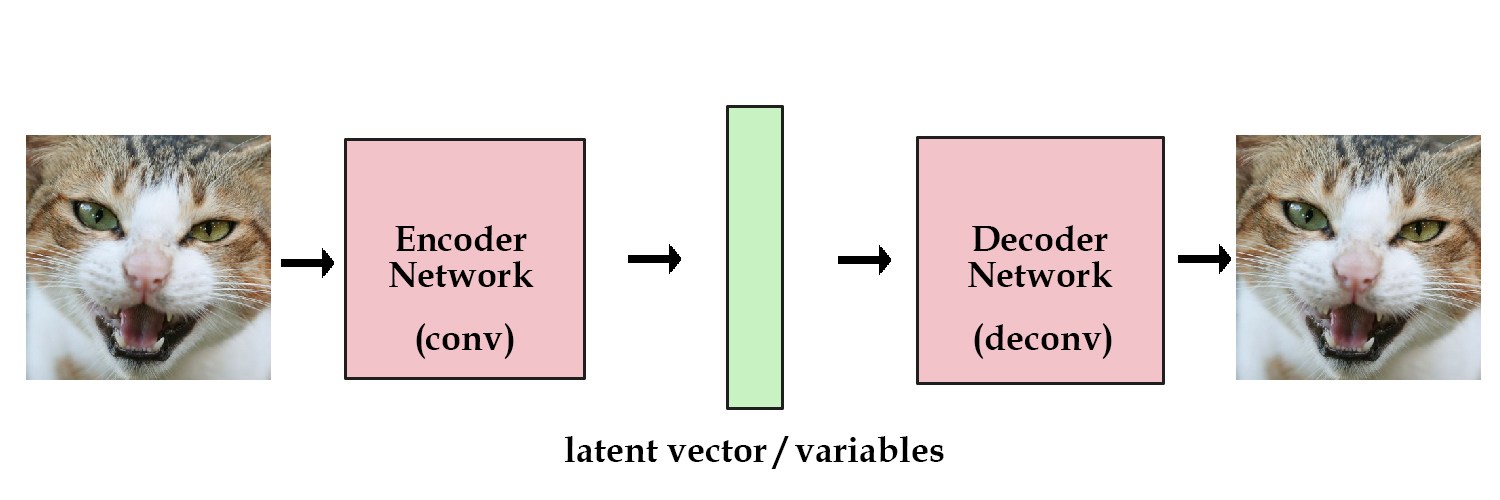[Updated 2018-11-25]

• Downsides of GANs:
• Images come from arbitrary noise, so must search over large space to find good initial noise values
• No constraints, only “real” or “fake”. Can lead to degenerate cases where no actual object in image.
• Autoencoder solves these problems
• AE adds an encoder network that encodes latent vector/variables, then passes to decoder.• If we save the encoded vector of an image, we can reconstruct it later by passing it into the decoder portion.
• Can’t generate anything like this though – need an existing image to generate latent vector
• Solution: add a constraint on encoding network forcing it to generate latent vectors following a unit gaussian distribution
• This makes it variational
• Then, to generate stuff, you can just sample from the unit gaussian distribution and pass it directly to the decoder, which will in theory produce a novel result.
• Two losses in network:
• Generative (MSE measuring reconstruction accuracy)
• Latent (KL divergence that measures how closely latent variables match unit gaussian)
• Think of a latent variable as a transfer of data
• Let’s say you were given a bunch of pairs of real numbers between [0, 10], along with a name. For example, 5.43 means apple, and 5.44 means banana. When someone gives you the number 5.43, you know for sure they are talking about an apple. We can essentially encode infinite information this way, since there’s no limit on how many different real numbers we can have between [0, 10].

However, what if there was a gaussian noise of one added every time someone tried to tell you a number? Now when you receive the number 5.43, the original number could have been anywhere around [4.4 ~ 6.4], so the other person could just as well have meant banana (5.44).

The greater standard deviation on the noise added, the less information we can pass using that one variable.

Now we can apply this same logic to the latent variable passed between the encoder and decoder. The more efficiently we can encode the original image, the higher we can raise the standard deviation on our gaussian until it reaches one.

This constraint forces the encoder to be very efficient, creating information-rich latent variables. This improves generalization, so latent variables that we either randomly generated, or we got from encoding non-training images, will produce a nicer result when decoded.

• Because of encoder-decoder scheme, can compare generated images directly to the originals
• Downside to VAE: mean squared error produces fuzzy images

• Combine VAE and GAN: use adversarial network as a metric for training decoder? Hm beyond current mental capacity